• 高斯函数Gram-Schmidt正交归一化介绍Gram-Schmidt OrthonormalizationGram-Schmidt Orthonormalization 介绍Gram-Schmidt Orthonormalization 首先介绍一下任意函数的Gram-Schmidt Orthonormalization操作，然后将其...
高斯函数Gram-Schmidt正交归一化介绍Gram-Schmidt OrthonormalizationGram-Schmidt OrthonormalizationGram-Schmidt Orthonormalization应用在高斯基函数上
介绍Gram-Schmidt Orthonormalization
首先介绍一下任意函数的Gram-Schmidt Orthonormalization操作，然后将其应用到高斯函数上
Gram-Schmidt Orthonormalization
“Gram-Schmidt orthogonalization, also called the Gram-Schimdt process, is a procedure which takes a nonorthogonal set of linearly independent functions and constructs an orthongonal basis over an arbitary interval with respect to an arbitary weighting function $w(x)$.”
Given an original set of linearly independent functions $\{u_n\}_{n=0}^{\infty}$, let $\{\psi_n\}_{n=0}^{\infty}$ denote the orthogonalized (but not normalized) functions,  $\{\phi_n\}_{n=0}^{\infty}$ denote the orthornomarlized functions, and define
$\psi_0(x)\equiv u_0(x) \\ \phi_0(x)\equiv {\psi_0(x)\over{\sqrt {\phi^2_0(x)w(x)dx}}} \\ \phi_1(x) \equiv u_1(x)+a_{10}\phi_0(x) \\ \int{\phi^2_0(x)}wdx=1 a_{10}=-\int u_1\phi_0wdx \\ \psi_1=u_1(x)-[\int u_1\phi_0wdx]\phi_0(x) \\ \phi_1(x)={\psi_1(x)\over{\sqrt {\phi^2_1(x)w(x)dx}}} \\ \phi_i(x)={\psi_i(x)\over{\sqrt {\phi^2_i(x)w(x)dx}}} \\ \psi_i(x)=u_i+a_{i0}\phi_0+a_{i1}\phi_1 +\ldots+ a_{i,i-1}\phi_{i-1} \\ a_{ij}=-\int u_i\phi_jwdx$
If the functions are normalized to $N_j$ instead of 1, then
$\int_{a}^{b}[\phi_j(x)]^2wdx=N_j^2 \\ \phi_j(x)=N_i{\psi_i(x)\over\sqrt{\psi^2_i(x)wdx}} a_{ij}=-{{\int u_i\phi_jwdx}\over{N^2_j}}$
Gram-Schmidt Orthonormalization应用在高斯基函数上
高斯基函数的径向函数为$R_l(r)=r^{l}e^{(-\alpha r^2)}$, 积分区间为$r\in(0,\infty)$,weight functions是$w(r)=r^2$,下面以两个高斯基函数为例进行Gram-Schmidt Orthonormalization：
$u_0(r)=r^{l}e^{(-\alpha_1 r^2)}\\ u_1(r)=r^{l}e^{(-\alpha_2 r^2)}\\ w(r)=r^2\\ \psi_0(r)\equiv u_0(r) = r^{l}e^{(-\alpha_1 r^2)}\\ \phi_o(x)\equiv {\psi_0(x)\over{\sqrt {\psi^2_0(r)w(r)dr}}} \\ ={r^{l}e^{(-\alpha_1 r^2)}\over {\sqrt {r^{2l}e^{(-2\alpha_1 r^2)}\cdot r^2dr}}} \\$


展开全文• 我试图从文本文件中读取数据集,提取3个主要参数,并将它们放在单独的列表中,并在分配高斯分布函数后对参数列表(a,b,c)应用规范。为了取得好成绩我把正数和负数分开每个参数的列表并对其应用高斯分布函数分别地挑选:...
我试图从文本文件中读取数据集,提取3个主要参数,并将它们放在单独的列表中,并在分配高斯分布函数后对参数列表(a,b,c)应用规范化。为了取得好成绩我把正数和负数分开每个参数的列表并对其应用高斯分布函数分别地挑选:意思是价值负数作为真实最小值意思是价值正数作为真实最大值而不是直接在这些参数的主列表中找到可能重复的最小最大值几次因为他们不合意置信区间. 如下图所示,所有步骤可分为4步完成,其中包括2个24x20矩阵图,图中数据帧中的一列由于数据的巨大回归和散射以及不必要的噪声(使其无法解释)而不清晰: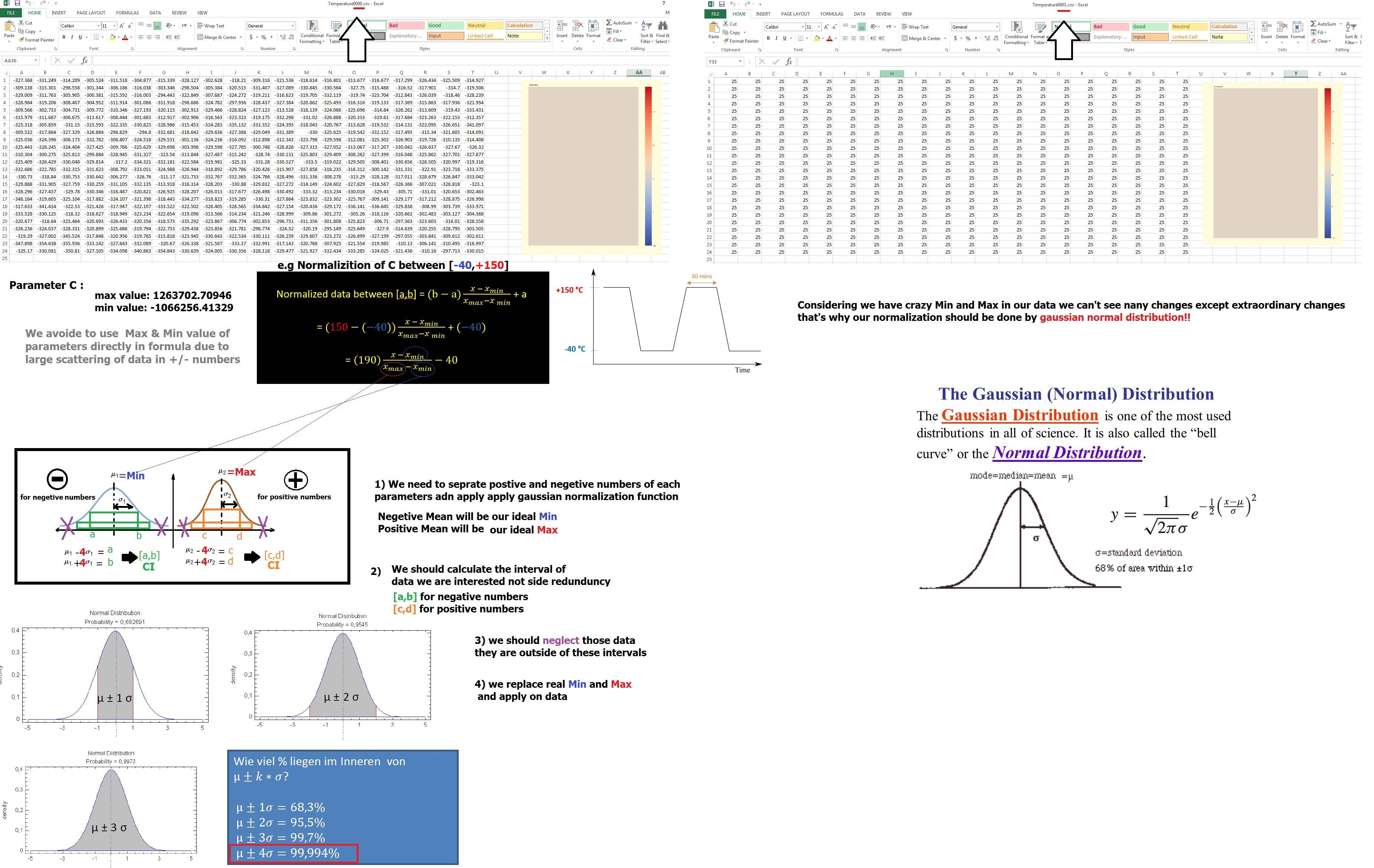考虑到像[a,b]这样的区间之间的标准化没有确定的答案,我根据它的公式定义函数:def normalize(value, min_value, max_value, min_norm, max_norm):new_value = ((max_norm - min_norm)*((value - min_value)/(max_value - min_value))) + min_normreturn new_value所以我的剧本如下:import numpy as npimport pandas as pdimport matplotlib.pyplot as pltimport scipyimport warningswarnings.filterwarnings("ignore",category =RuntimeWarning)dft = pd.read_csv('D:/me.txt', header=None)id_set = dft[dft.index % 4 == 0].astype('int').valuesA = dft[dft.index % 4 == 1].valuesB = dft[dtf.index % 4 == 2].valuesC = dft[dft.index % 4 == 3].valuesdata = {'A': A[:,0], 'B': B[:,0], 'C': C[:,0]} # arrays#main_data contains all the datadf = pd.DataFrame(data, columns=['A','B','C'], index = id_set[:,0])df  = df.replace([np.inf, -np.inf], np.nan).astype(np.float64)df  = df.fillna(0.012345)def normalize(value, min_value, max_value, min_norm, max_norm):new_value = ((max_norm - min_norm)*((value - min_value)/(max_value - min_value))) + min_normreturn new_valuedef createpositiveandnegativelist(listtocreate):l_negative = []l_positive = []for value in listtocreate:if (value < 0):l_negative.append(value)elif (value > 0):l_positive.append(value)#print(t_negative)#print(t_positive)return l_negative,l_positivedef calculatemean(listtocalculate):return sum(listtocalculate)/len(listtocalculate)def plotgaussianfunction(mu,sigma):s = np.random.normal(mu, sigma,1000)abs(mu - np.mean(s))<0.01abs(sigma - np.std(s,ddof=1))<0.01#count, bins, ignored = plt.hist(s,30,density=True)#plt.plot(bins, 1/(sigma * np.sqrt(2 * np.pi)) * np.exp(-(bins-mu)**2/(2*sigma**2)),linewidth=2, color= 'r')#plt.show()returndef plotboundedCI(s, mu, sigma, lists):plt.figure()count, bins, ignored = plt.hist(s,30,density=True)plt.plot(bins, 1/(sigma * np.sqrt(2 * np.pi)) * np.exp(-(bins-mu)**2/(2*sigma**2)),linewidth=2, color= 'r')#confidential interval calculationci = scipy.stats.norm.interval(0.68, loc = mu, scale = sigma)#confidence interval for left lineone_x12, one_y12 = [ci,ci], [0,3]#confidence interval for right linetwo_x12, two_y12 = [ci,ci], [0,3]plt.title("Gaussian 68% Confidence Interval", fontsize=12, color='black', loc='left', style='italic')plt.plot(one_x12, one_y12, two_x12, two_y12, marker = 'o')plt.show()#get sure about avoiding the outliers of CIresults = []for value in lists:if(ci< value results.append(value)else:#print("NOT WANTED: ",value)passreturn resultsc_negative, c_positive = createpositiveandnegativelist(C)b_negative, b_positive = createpositiveandnegativelist(B)a_negative, a_positive = createpositiveandnegativelist(A)#get max and min valuesa_min = main_data['A'].min()a_max = main_data['A'].max()b_min = main_data['B'].min()b_max = main_data['B'].max()c_min = main_data['C'].min()c_max = main_data['C'].max()print ("\ntmp Negative Min",c_min)print ("\n tmp Positive Max",c_max)#calculating the mean valuec_p_mean = calculatemean(c_positive)b_p_mean = calculatemean(b_positive)a_p_mean = calculatemean(a_positive)c_n_mean = calculatemean(c_negative)b_n_mean = calculatemean(b_negative)a_n_mean = calculatemean(a_negative)print ("\ntmp Negative Mean",c_n_mean)print ("\n tmp Positive Mean",c_p_mean)#calculating the sigma valuec_sigma_Negative = np.std(c_negative)c_sigma_Positive = np.std(c_positive)b_sigma_Negative = np.std(c_negative)b_sigma_Positive = np.std(c_positive)a_sigma_Negative = np.std(c_negative)a_sigma_Positive = np.std(c_positive)#plot the gaussian function with histogramsplotgaussianfunction(c_p_mean, c_sigma_Positive)plotgaussianfunction(c_n_mean, c_sigma_Negative)plotgaussianfunction(b_p_mean, b_sigma_Positive)plotgaussianfunction(b_n_mean, b_sigma_Negative)plotgaussianfunction(a_p_mean, a_sigma_Positive)plotgaussianfunction(a_n_mean, a_sigma_Negative)#normalizationc_p_s = np.random.normal(c_p_mean, c_sigma_Positive, 1000)c_n_s = np.random.normal(c_n_mean, c_sigma_Negative, 1000)b_p_s = np.random.normal(b_p_mean, b_sigma_Positive, 1000)b_n_s = np.random.normal(b_n_mean, b_sigma_Negative, 1000)a_p_s = np.random.normal(a_p_mean, a_sigma_Positive, 1000)a_n_s = np.random.normal(a_n_mean, a_sigma_Negative, 1000)#histograms minus the outliersc_p_results = plotboundedCI(c_p_s, c_p_mean, c_sigma_Positive, c_positive)c_n_results = plotboundedCI(c_n_s, c_n_mean, c_sigma_Negative, c_negative)b_p_results = plotboundedCI(b_p_s, b_p_mean, b_sigma_Positive, b_positive)b_n_results = plotboundedCI(b_n_s, b_n_mean, b_sigma_Negative, b_negative)a_p_results = plotboundedCI(a_p_s, a_p_mean, a_sigma_Positive, a_positive)a_n_results = plotboundedCI(a_n_s, a_n_mean, a_sigma_Negative, a_negative)#next iteration create all plots, change the number of cyclesfor i in df:if 'C' in i:min_nor = -40max_nor = 150#Applying normalization for C between [-40,+150]new_value3 = normalize(df['C'].iloc[j:j+480], c_n_mean, c_p_mean, -40, 150)n_cbar_kws = {"ticks":[-40,150,-20,0,25,50,75,100,125]}df3 = print_df(mkdf(new_value3))df3.to_csv(f'{i}/norm{i}{count}.csv', header=None, index=None)else:#Applying normalizayion for A,B between    [-1,+1]new_value1 = normalize(df['A'].iloc[j:j+480], a_n_mean, a_p_mean, -1, 1)new_value2 = normalize(df['B'].iloc[j:j+480], b_n_mean, b_p_mean, -1, 1)n_cbar_kws = {"ticks":[-1.0,-0.75,-0.50,-0.25,0.00,0.25,0.50,0.75,1.0]}df1 = print_df(mkdf(new_value1))df2 = print_df(mkdf(new_value2))df1.to_csv(f'{i}/norm{i}{count}.csv', header=None, index=None)df2.to_csv(f'{i}/norm{i}{count}.csv', header=None, index=None)'''for i in df:if i=='C':#Applying normalizayion for C between [-40,+150]data['C']  = normalize(df[i].values, c_n_mean, c_p_mean, -40, 150)elif i=='A':#Applying normalization for A , B between [-1,+1]data['A'] = normalize(df[i].values, a_n_mean, a_p_mean, -1, 1)else:data['B'] = normalize(df[i].values, b_n_mean, b_p_mean, -1, 1)'''norm_data = pd.DataFrame(data, index = id_set[:,0])print(norm_data)norm_data.to_csv('norm.csv')问题是:γ问题I:我已经避免了运行时警告错误,但仍然有以下错误,我不知道如何解决,包括值错误:缩放<0可能是由于正负数的分离。γ问题二:在标准化之后,可能会发生超过10个周期(每个周期有480个值),我已经意识到考虑对两个-/+数字应用高斯函数,然后在[a,b]之间使用最小、最大的标准化,在这种情况下,仍有一些值超出范围,如下图所示或者列'c'超出了[-40,+150],这太奇怪了,超出了我的预期!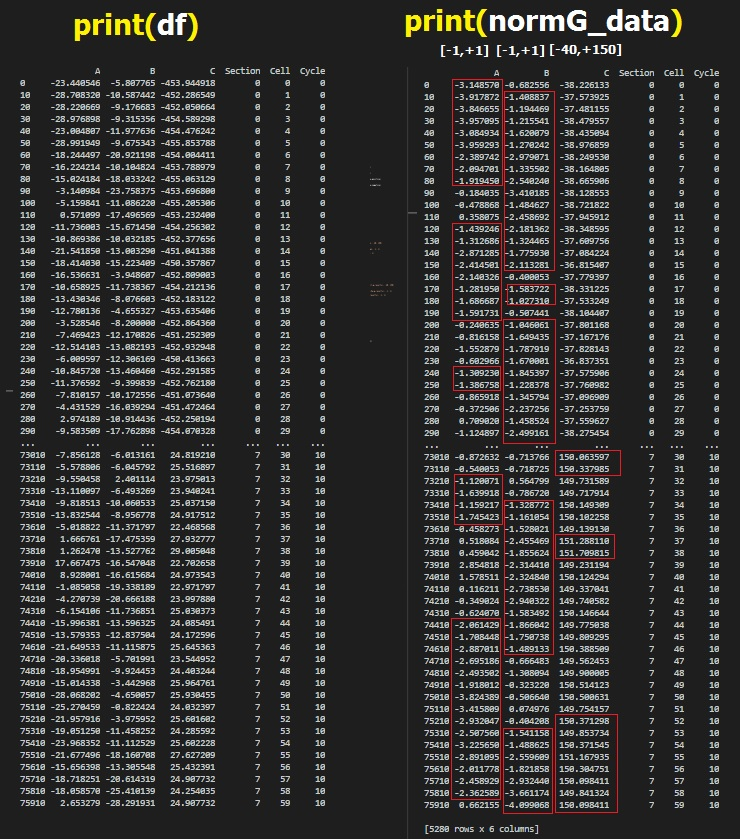希望有人对错误的解决方案有一个好的想法,或者使用高斯分布函数应用规范化的更好方法,谢谢您的关注。注1:我有一些缺少数据(NAN或INF)在我的值列表中,这些值已经被0替换了(确切地说是0.012345)!但是考虑到当我的参数列表中没有丢失的值时,代码就可以工作了!注2:我面临着这样的错误:ValueError: max must be larger than min in range parameter.对于count, bins, ignored = plt.hist(s,30,density=True)和plotgaussianfunction(t_p_mean, t_sigma_Positive)我认为是关于这个条件的:abs(sigma - np.std(s,ddof=1)) < 0.01因为我也有类似的错误ValueError: scale < 0对于s = np.random.normal(mu, np.abs(sigma) ,1000)我已经问过了here我提供了3个周期的数据集样本:dataset我还提供了11个周期的另一个数据集:dataset
展开全文• 高斯金字塔 – 用来对图像进行降采样 拉普拉斯金字塔 – 用来重建一张图片根据它的上层降采样图片 高斯金字塔是从底向上，逐层降采样得到。 降采样之后图像大小是原图像MxN的M/2 x N/2 ,就是对原图像删除偶数行与列...
图像金字塔：
高斯金字塔 – 用来对图像进行降采样
拉普拉斯金字塔 – 用来重建一张图片根据它的上层降采样图片
高斯金字塔是从底向上，逐层降采样得到。
降采样之后图像大小是原图像MxN的M/2 x N/2 ,就是对原图像删除偶数行与列，即得到降采样之后上一层的图片。
高斯金子塔的生成过程分为两步：

对当前层进行高斯模糊
删除当前层的偶数行与列
即可得到上一层的图像，这样上一层跟下一层相比，都只有它的1/4大小。

上采样与降采样
上采样,生成图像是原图在宽高各放大两倍
pyrUp(Mat src, Mat dst, Size(src.cols2, src.rows2));
降采样,生成图像是原图在宽高各缩小1/2
pyrDown(Mat src, Mat dst, Size(src.cols/2, src.rows/2));
高斯差分
Difference of Gaussian(DOG)是高斯函数的差分。将两幅图像在不同参数下的高斯滤波结果相减，得到DoG图。步骤：
处理一幅图像在不同高斯参数下的DoG
用两个不同的5x5高斯核对图像进行卷积，然后再相减的操作。重复三次得到三个差分图A,B,C。
根据DoG求角点
计算出的A,B,C三个DOG图中求图B中是极值的点。图B的点在当前由A,B,C共27个点组成的block中是否为极大值或者极小值。若满足此条件则认为是角点。
Mat gray, g1, g2;
cvtColor(src, gray, CV_BGR2GRAY);
GaussianBlur(gray, g1, Size(3, 3), 0, 0);
GaussianBlur(g1, g2, Size(3, 3), 0, 0);
subtract(g1, g2, dst, Mat());
归一化函数
void normalize(InputArray src,OutputArraydst, double alpha = 1, double beta = 0, intnorm_type = NORM_L2, int dtype = -1, InputArray mask = noArray() )
参数说明:
src——输入数组
dst——输出数组，支持原地运算
alpha——range normalization模式的最小值
beta ——range normalization模式的最大值，不用于norm normalization(范数归一化)模式。
normType——归一化的类型，可以有以下的取值：
NORM_MINMAX:数组的数值被平移或缩放到一个指定的范围，线性归一化，一般较常用。
NORM_INF:此类型的定义没有查到，根据OpenCV 1的对应项，可能是归一化数组的C-范数(绝对值的最大值)
NORM_L1 : 归一化数组的L1-范数(绝对值的和)
NORM_L2: 归一化数组的(欧几里德)L2-范数
dtype——dtype为负数时，输出数组的type与输入数组的type相同；否则，输出数组与输入数组只是通道数相同，而tpye=CV_MAT_DEPTH(dtype).
操作掩膜，用于指示函数是否仅仅对指定的元素进行操作。
threshold(gray, dst, threshold_value, threshold_max, type_value);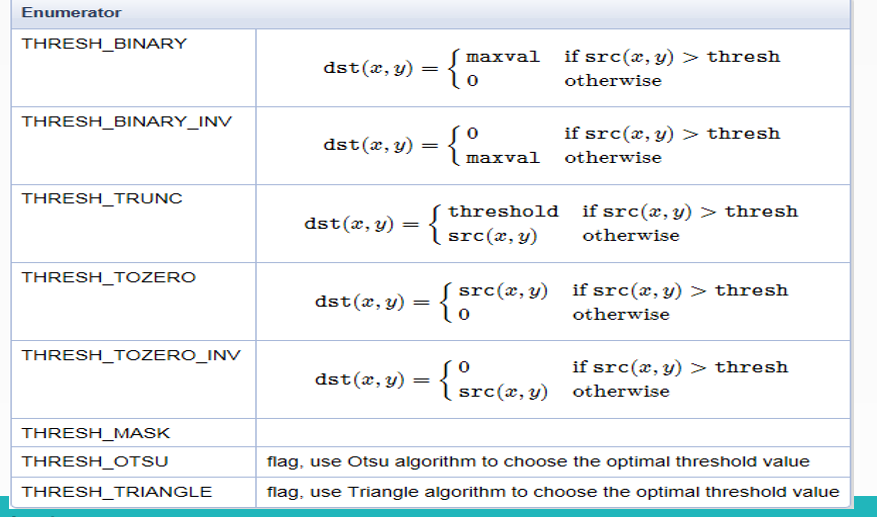边缘填充
在卷积开始之前增加边缘像素，填充的像素值为0或者RGB黑色，比如3x3在
四周各填充1个像素的边缘，这样就确保图像的边缘被处理，在卷积处理之
后再去掉这些边缘。openCV中默认的处理方法是： BORDER_DEFAULT，此外
常用的还有如下几种：

BORDER_CONSTANT – 填充边缘用指定像素值
BORDER_REPLICATE – 填充边缘像素用已知的边缘像素值。
BORDER_WRAP – 用另外一边的像素来补偿填充

给图像添加边缘API
copyMakeBorder（

Mat src, // 输入图像
Mat dst, // 添加边缘图像
int top, // 边缘长度，一般上下左右都取相同值，
int bottom,
int left,
int right,
int borderType // 边缘类型
Scalar value
）

参考文献：51CTO学院讲义


展开全文• 目录高斯变量基础高斯分布概率密度函数性质复高斯分布概率密度函数应用零均值循环对称复高斯随机变量零均值化卡方分布补充归一化标准化 高斯变量基础 高斯分布 概率密度函数 性质 复高斯分布 若复高斯分布Z=X+iY,...
目录高斯变量基础高斯分布概率密度函数性质复高斯分布概率密度函数应用零均值循环对称复高斯随机变量零均值化卡方分布补充归一化标准化加性高斯白噪声
高斯变量基础
高斯分布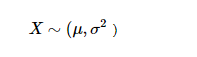概率密度函数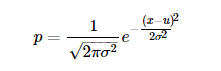性质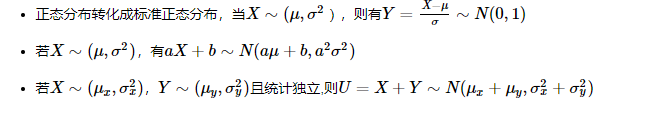复高斯分布
若复高斯分布Z=X+iY, 且满足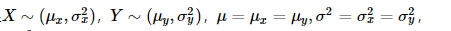则有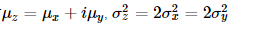概率密度函数注：复高斯随机变量的密度函数，分母已经没有根号
应用
零均值循环对称复高斯随机变量
特殊的，当μ=μx=μy=0时，Z称为零均值循环对称复高斯随机变量（zero mean circle symmetric complex gaussian,ZMCSCG）,σ2称为每个实数维度上的方差。
以上分析得出复高斯随机变量与每一实数维度高斯随机变量的关系
零均值化
将每个像素的值减去训练集上所有像素值的平均值，比如已计算得所有像素点的平均值为128，所以减去128后，现在的像素值域即为[-128,127]，即满足均值为零。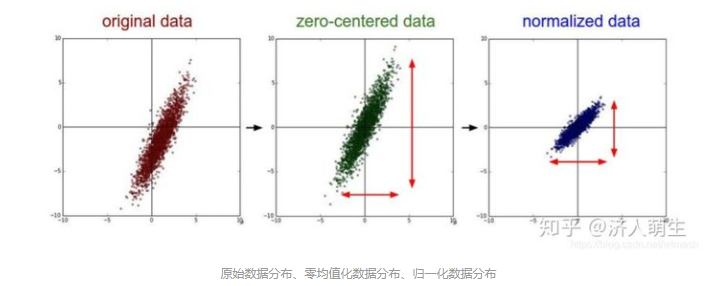卡方分布
设X1,X2,X3,…,i.i.d∼N(0,1), 令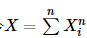, 则X是服从自由度为n的χ2分布，记为X∼χ2(n)
卡方分布为特殊的Gamma分布，服从参数为G(n/2,1/2)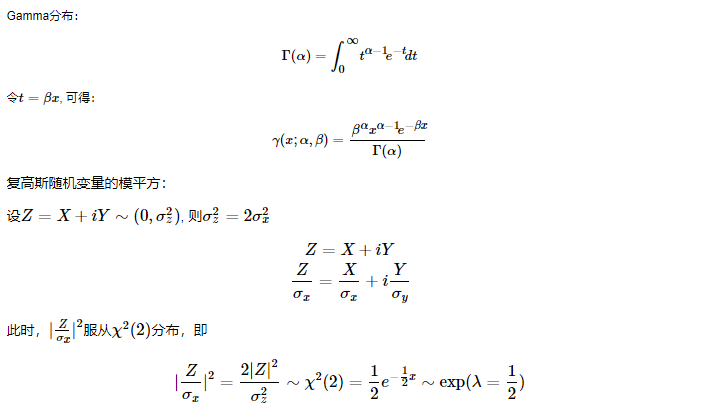注意：
复高斯随机变量概率表达式的分母
复高斯随机变量模平方的分布与卡方分布、指数分布、Gamma分布之间的关系

补充
归一化、标准化、零均值化核心思想：平移+缩放
归一化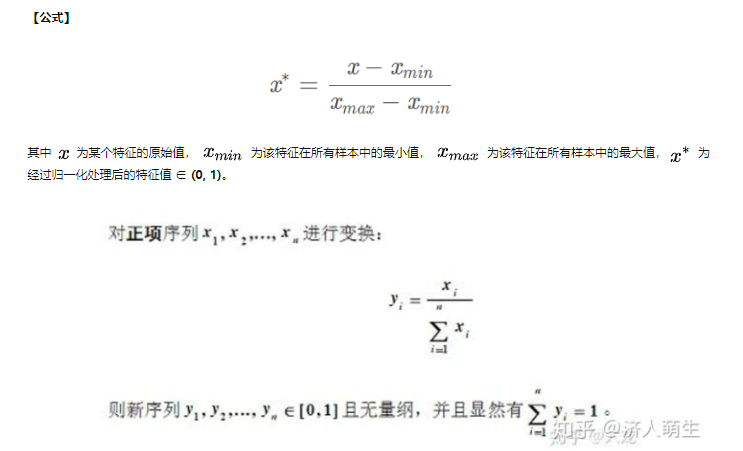【作用】将某个特征的值映射到[0,1]之间，消除量纲对最终结果的影响，使不同的特征具有可比性，使得原本可能分布相差较大的特征对模型有相同权重的影响，提升模型的收敛速度，深度学习中数据归一化可以防止模型梯度爆炸。
标准化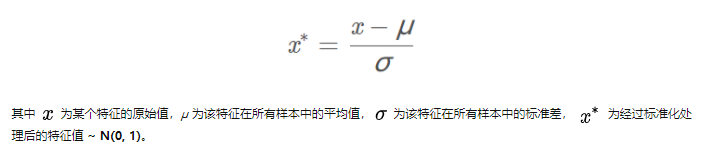【作用】将原值减去均值后除以标准差，使得得到的特征满足均值为0，标准差为1的正态分布，使得原本可能分布相差较大的特征对模型有相同权重的影响。举个例子，在KNN中，需要计算待分类点与所有实例点的距离。假设每个实例（instance）由n个features构成。如果选用的距离度量为欧式距离，数据预先没有经过归一化，那些绝对值大的features在欧式距离计算的时候起了决定性作用。
从经验上说，标准化后，让不同维度之间的特征在数值上有一定比较性，得出的参数值的大小可以反应出不同特征对样本label的贡献度，可以大大提高分类器的准确性。
【二者比较】具体应该选择归一化还是标准化呢，如果把所有维度的变量一视同仁，
①在计算距离中发挥相同的作用，应该选择标准化，标准化更适合现代嘈杂大数据场景。
②如果想保留原始数据中由标准差所反映的潜在权重关系，或数据不符合正态分布时，选择归一化。

参考https://zhuanlan.zhihu.com/p/183591302
https://www.cnblogs.com/zwwangssd/p/12540280.html

加性高斯白噪声
高斯是指概率分布是正态函数，白噪声是指他的二阶矩不相关，一阶矩为常数，是指先后信号在时间上的相关性。高斯白噪声是研究信道加性噪声的理想模型，通信中的主要噪声源—热噪声就属于这类噪声。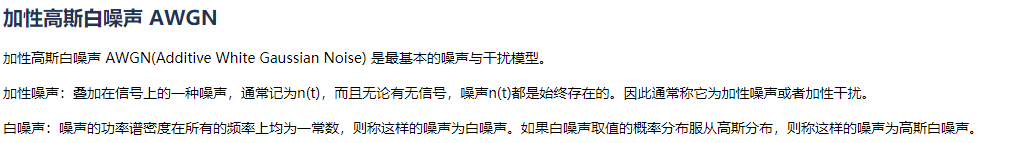展开全文• 比如使用5*5的模板,(sigma=1.4)得到的是归一化的高斯滤波模板，高斯函数最开始的公式计算出来的是小数，因为小数计算过程更缓慢，所以为了提高运算效率，将其写成整数模板的形式，另一方面小数模板在实际的像素上面...
• 数据归一化（或者标准化，注意归一化和标准化不同）的...有些模型伸缩与原来等价，如：LR则不用归一化，但是实际中往往通过迭代求解模型参数，如果目标函数太扁（想象一下很扁的高斯模型）迭代算法会发生不收敛的情...
• 有些模型伸缩有与原来等价，如：LR则不用归一化，但是实际中往往通过迭代求解模型参数，如果目标函数太扁（想象一下很扁的高斯模型）迭代算法会发生不收敛的情况，所以最好进行数据归一化。 ...机器学习
• 1、线性函数归一化(Min-Max scaling) 线性函数将原始数据线性化的方法转换到[0 1]的范围，归一化公式如下： ...该种归一化方式要求原始数据的分布可以近似为高斯分布，否则归一化的效果会变得
• ## 归一化的本质原因

千次阅读 2018-11-21 10:43:03
数据归一化（或者标准化，注意归一化和标准化不同）...有些模型伸缩有与原来等价，如：LR则不用归一化，但是实际中往往通过迭代求解模型参数，如果目标函数太扁（想象一下很扁的高斯模型）迭代算法会发生不收敛的情...
• %归一化处理 另一个是 k1=8; k2=8; r=161;%定义模板的大小 alf=1600; %标准差 nn=floor((r+1)/2); % 计算中心 尺度c=80效果做好 for i=1:r for j=1:r b(i,j) =exp(-((i-nn)^2+(j-nn)^2)/(k1*alf))/...retinex
• ## Matlab归一化方法

万次阅读 2018-05-10 10:41:09
1，标准归一化。 将原始数据集归一化为均值为0、方差1的数据集，归一化公式如下： x∗=x−μδ　其中μ为所有样本数据的均值，δ为所有样本数据的标准差。 这种方式要求原始数据集的分布近似为正态（高斯）分布。...
• 本教程教您怎样使用各种线性滤波器对图像进行平滑处理，相关OpenCV函数如下: blurGaussianBlurmedianBlurbilateralFilter 原理 Note   以下原理来源于Richard Szeliski 的著作 Computer Vision: ...
• ## 数据归一化

千次阅读 2016-05-11 17:21:22
- 达到更好的模型训练效果（如BP算法中加速收敛）方法线性函数归一化如使用原始数据的最小、最大值将原始数据变换到[0,1]范围内 0均值标准化（Z-scorestandardization）将原始数据转化为均值为0，方差为1的数据集...数据预处理
• Atitit 图像处理 平滑 也称 模糊, 归一化块滤波、高斯滤波、中值滤波、双边滤波）    是一项简单且使用频率很高的图像处理方法   用途 去噪 去雾   各种线性滤波器对图像进行平滑处理，相关OpenCV...
• OpenCV 高斯模糊函数的调用测试1.图片读入，彩色转灰度2.计算第一次高斯模糊3.计算第二次高斯模糊4.计算两次高斯模糊的差5.结果归一化 1.图片读入，彩色转灰度 2.计算第一次高斯模糊 3.计算第二次高斯模糊 4.计算...
• Atitit 图像处理平滑也称模糊,归一化块滤波、高斯滤波、中值滤波、双边滤波） 是一项简单且使用频率很高的图像处理方法 用途去噪 去雾 各种线性滤波器对图像进行平滑处理，相关OpenCV函数如下: 归一化块...
• 其主要实现函数为SlamSystem::trackFrame这个函数的代码主要分为如下几个步骤实现：构造新的图像帧：把当前图像构建为新的图像帧更新参考帧：如果当前参考帧不是最近的关键帧，则更新参考帧初始位姿：把上一帧与...
• 如果我们想要正确的激活单位高斯函数，我们可以取目前处理的批数据的均值，利用均值和方差来进行归一化，如果我们要在训练的每一层都保持良好的高斯分布，就需要进行批量归一化。而且需要它能够微分，这样才能在梯度...
• BN的意义：在激活函数之前将输入归一化高斯分布，控制到激活函数的敏感区域，避免激活函数的梯度饱和导致梯度消失，增加学习效率 （1）加速收敛（2）控制过拟合，可以少用或不用Dropout和正则（3）降低网络对初始...
• 自写函数–高斯滤波算法 高斯噪声 高斯噪声是指它的概率密度函数服从高斯(即正态分布)分布 一维高斯函数 二维高斯函数 ...高斯滤波器对于抑制服从正态分布的噪声非常有效 ... 计算最终的权重矩阵（归一化） ...算法
• 支持向量机，高斯函数背景介绍核心思想公式推导及主要函数函数核心思想公式推导及函数名词介绍希尔伯特空间（Hilbert space）内积归一化和标准化内积空间（pre-Hilbert space/欧几里得空间）范数范数和距离...机器学习 支持向量机 深度学习
• 批量归一化（Batch Normalization），用于对神经元的输入进行归一化处理，使其符合高斯分布（不一定），解决饱和问题带来的梯度消失问题。 它一般被放置在全连接层或者卷积层之后，激活函数之前。如下图所示。其中右...深度学习 python 机器学习 人工智能
• 如果我们想要正确的激活单位高斯函数，我们可以取目前处理的批数据的均值，利用均值和方差来进行归一化，如果我们要在训练的每一层都保持良好的高斯分布，就需要进行批量归一化。而且需要它能够微分，这样才能在梯度...
• 为了使重建的图像能更好地保持边缘细节，采用各向异性高斯函数作为适用度函数，并将改进的自适应归一化卷积超分辨率重建算法应用于设计的多通道光学成像系统图像。由于各向异性高斯函数邻域的尺度和方向由提出的...
• 批次归一化（Batch normalization) 尤其使用于CNN 主要作用是将数据拉回到均值为零方差为一的高斯正态模型下，以便位于激活函数的敏感范围。 会使用两个参数保证其非线性能力 详见深入理解Batch Normalization批标准...
• % gaussKernel():归一化二维高斯滤波核函数 % sig 对应于高斯函数定义中的σ % w 的大小为 m×m 其中m必须为奇数 %output1:从高斯函数采样得到的模板 %output2:模板除以第一个元素 %output1:取整后的模板 %outmatlab 卷积 算法 矩阵
• 其中一些是众所周知的（由随机湍流平移的平衡临界动力学模型和线性无源标量场模型A），但是又有一个新的非平衡状态（大学类）与重新归一化组方程的新非平凡不动点相关。 我们已经获得了系统中可能的缩放比例的相位......